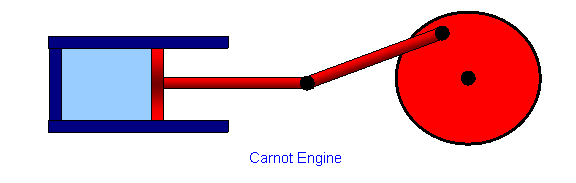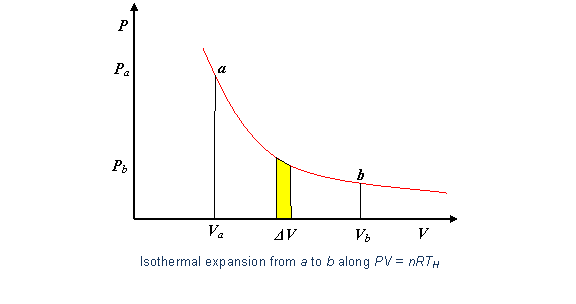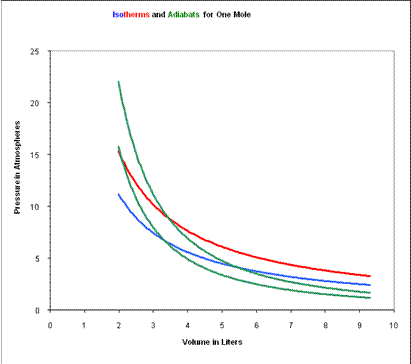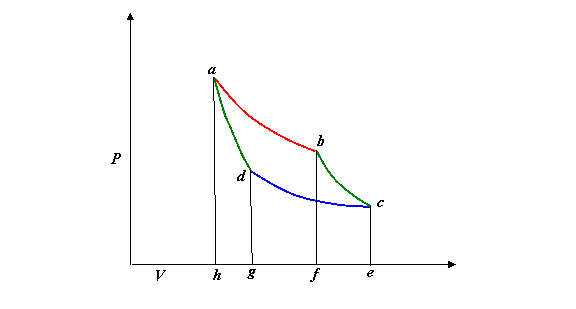$$\require{cancel}$$

# 1.7: The Carnot Engine

•• Contributed by Michael Fowler
• Beams Professor (Physics) at University of Virginia

### The Ultimate in Fuel Efficiency

All standard heat engines (steam, gasoline, diesel) work by supplying heat to a gas, the gas then expands in a cylinder and pushes a piston to do its work.  The catch is that the heat and/or the gas must somehow then be dumped out of the cylinder to get ready for the next cycle.

Our aim in this lecture is to figure out just how efficient such a heat engine can be: what’s the most work we can possibly get for a given amount of fuel?  We’ll examine here the simplest cyclical model: an ideal gas enclosed in a cylinder, with external connections to supply and take away heat, and a frictionless piston for the gas to perform (and to absorb) mechanical work:The efficiency question was first posed—and solved—by Sadi Carnot in 1820, not long after steam engines had become efficient enough to begin replacing water wheels, at that time the main power sources for industry.  Not surprisingly, perhaps, Carnot visualized the heat engine as a kind of water wheel in which heat (the “fluid”) dropped from a high temperature to a low temperature, losing “potential energy” which the engine turned into work done, just like a water wheel.

(Historical Note: actually, Carnot thought at the time that heat was a fluid—he believed in the Caloric Theory.  Remarkably, the naïve “potential energy of a caloric fluid” approach gives exactly the right answer for the efficiency of an ideal engine!  Carnot accepted that there was an absolute zero of temperature, from which he figured out that on being cooled to absolute zero, the caloric fluid would give up all its heat energy.  Therefore, if it falls only half way to absolute zero from its beginning temperature, it will give up half its heat, and an engine taking in heat at T and shedding it at ½T will be utilizing half the possible heat, and be 50% efficient.  Picture a water wheel that takes in water at the top of a waterfall, but lets it out halfway down.  So, the efficiency of an ideal engine operating between two temperatures will be equal to the fraction of the temperature drop towards absolute zero that the heat undergoes.  This turns out to be exactly correct, even though the reasoning is based on a false model.)

The water wheel analogy proved to be useful in another way: Carnot knew that the most efficient water wheels were those that operated smoothly, the water went into the buckets at the top from the same level, it didn’t fall into them through any height, and didn’t splash around.  In the idealized limit of a frictionless water wheel, with gentle flow on and off the wheel, such a machine would be reversible—if the wheel is run backwards by power supplied from the outside, so it raises water back up, it will take the same power that the wheel was itself delivering in normal operation. This idealized water wheel is clearly perfectly efficient, so the analogs of zero friction and gentle flow are what we need in the perfect heat engine.

Getting friction as low as possible is obviously necessary, but what, exactly, is “gentle flow” in the heat engine?  For the water wheel, it meant having water at the top flow smoothly into buckets at the same height, no wasteful drop that would lose potential energy with no gain.  For the heat engine, the analog is to have heat flow from the heat supply into the engine with no drop in temperature. In a real engine, there must of course be a slight drop in temperature for the heat to flow at all (just as there is for a real water wheel), but this has to be minimized.

So, as the heat is supplied and the gas expands, the temperature of the gas must stay the same as that of the heat supply (the “heat reservoir”): the gas is expanding isothermally.  Similarly, it must contract isothermally later in the cycle as it sheds heat.

To figure out the efficiency, we need to track the engine through a complete cycle, finding out how much work it does, how much heat is taken in from the fuel, and how much heat is dumped in getting ready for the next cycle.  You might want to look at the flashlet to get the picture at this point: the cycle has four steps, an isothermal expansion as heat is absorbed, followed by an adiabatic expansion, then an isothermal contraction as heat is shed, finally an adiabatic contraction to the original configuration.  We’ll take it one step at a time.

### Step 1: Isothermal Expansion

So the first question is: How much heat is supplied, and how much work is done,  as the gas expands isothermally?

Taking the temperature of the heat reservoir to be TH  (H for hot), the expanding gas follows the isothermal path $$PV = nRT_H$$ in the (P, V) plane.The work done by the gas in a small volume expansion $$\Delta V$$ is just $$P \Delta V$$, the area under the curve (as we proved in the last lecture).

Hence the work done in expanding isothermally from volume Va to Vb is the total area under the curve between those values, $work \space done \space isothermally \space = \int \limits_{V_a}^{V_b} PdV = \int \limits_{V_a}^{V_b} \dfrac {nRT_H}{V} dV = nRT_H ln \dfrac{V_b}{V_a}$

Since the gas is at constant temperature, $$T_H$$ there is no change in its internal energy during this expansion, so the total heat supplied must be $$nRT_H ln \dfrac{V_b}{V_a}$$, the same as the external work the gas has done.

In fact, this isothermal expansion is only the first step: the gas is at the temperature of the heat reservoir, hotter than its other surroundings, and will be able to continue expanding even if the heat supply is cut off.  To ensure that this further expansion is also reversible, the gas must not be losing heat to the surroundings.  That is, after the heat supply is cut off, there must be no further heat exchange with the surroundings, the expansion must be adiabatic

### Step 2: Adiabatic Expansion

By definition, no heat is supplied in adiabatic expansion, but work is done.

The work the gas does  in adiabatic expansion is like that of a compressed spring expanding against a force—equal to the work needed to compress it in the first place, for an ideal (and perfectly insulated) gas.  So adiabatic expansion is reversible.

To find the work the gas does expanding adiabatically from Vb to Vc, say, the Step 1 analysis above is repeated for the adiabat $$PV^{\gamma} = P_bV_b^{\gamma},$$ $work \space done \space adiabatically \space W_{adiabat} = \int \limits_{V_b}^{V_a} PdV = P_bV_b^{\gamma} \int \limits_{V_b}^{V_c} \dfrac {dV}{V^{\gamma}} = P_bV_b^{\gamma} \dfrac {V_c^{1-\gamma} -V_b^{1-\gamma}}{1-\gamma}.$

Again, this work equals the area under the curve from b to c in the (P, V) plane.

Now $$P_cV_c^{\gamma} = P_bV_b^{\gamma}$$ so the expression can be written more neatly: $W_{adiabat} = \dfrac {P_cV_c - P_bV_b}{1-\gamma}$

This is a useful expression for the work done since we are plotting in the (P, V) plane, but note that from the gas law $$PV = nRT$$ the numerator is just $$nR(T_c - T_b)$$, and from this $$W_{adiabat} = nC_v (T_c -T_b)$$, as of course it must be—this is the loss of internal energy by the gas on expanding against the external pressure.

### Steps 3 and 4: Completing the Cycle

We’ve looked in detail at the work a gas does in expanding as heat is supplied (isothermally) and when there is no heat exchange (adiabatically).  These are the two initial steps in a heat engine, but it is necessary for the engine to get back to where it began, for the next cycle.  The general idea is that the piston drives a wheel (as in the diagram at the beginning of this lecture), which continues to turn and pushes the gas back to the original volume.

But it is also essential for the gas to be as cold as possible on this return leg, because the wheel is now having to expend work on the gas, and we want that to be as little work as possible—it’s costing us.  The colder the gas, the less pressure the wheel is pushing against.

To ensure that the engine is as efficient as possible, this return path to the starting point $$(P_a,V_a)$$ must also be reversible.  We can’t just retrace the path taken in the first two legs, that would take all the work the engine did along those legs, and leave us with no net output.  Now the gas cooled during the adiabatic expansion from b to c, from TH to TC, say, so we can go some distance back along the reversible colder isotherm TC.  But this won’t get us back to $$(P_a,V_a)$$, because that’s on the TH isotherm.  The simplest option—the one chosen by Carnot—is to proceed back along the cold isotherm to the point where it intersects the adiabat through a, then follow that adiabat back to a.  That route keeps the gas as cold as possible for as long as possible, minimizing the external work needed to get it back to the original state.

To picture the Carnot cycle in the (P, V) plane, recall from the previous lecture the graph showing two isotherms and two adiabats:Carnot’s cycle is around that curved quadrilateral having these four curves as its sides.

Let us redraw this, slightly less realistically but more conveniently:### Efficiency of the Carnot Engine

In a complete cycle of Carnot’s heat engine, the gas traces the path abcd.  The important question is: what fraction of the heat supplied from the hot reservoir (along the red top isotherm) is turned into mechanical work?  This fraction is called the efficiency of the engine.

The work output along any curve in the (P, V) plane is just $$\int PdV$$, the area under the curve, but it will be negative if the volume is decreasing!  So the work done by the engine during the hot isothermal segment is the area abfh, then the adiabatic expansion adds the area bcef, but as the gas is compressed back, the wheel has to do work on the gas equal to the area cdge as heat is dumped into the cold reservoir, then dahg as the gas is recompressed to the starting point.

The bottom line is that:

the total work done by the gas is the area of the circuit abcd

that is, the area of the curved “parallelogram” in the  (P, V) graph above.

We could compute this area by finding $$\int PdV$$ for each segment, but that is unnecessary—on completing the cycle, the gas is back to its initial temperature, so has the same internal energy.

Therefore, the work done by the engine must be just the difference between the heat supplied at TH and that dumped at TC.

Now the heat supplied along the initial hot isothermal path ab  is equal to the work done along that leg, (from the paragraph above on isothermal expansion): $Q_H = nRT_H ln \dfrac{V_b}{V_a}$

and the heat dumped into the cold reservoir along cd is $Q_c = nRT_c ln \dfrac{V_c}{V_d}.$

The difference between these two is the net work output. This can be simplified using the adiabatic equations for the other two sides of the cycle: $T_HV_b^{\gamma-1} = T_cV_c^{\gamma -1}$ $T_HV_a^{\gamma-1} = T_cV_d^{\gamma-1}$

Dividing the first of these equations by the second,$\left( \dfrac{V_b}{V_a} \right) = \left( \dfrac{V_c}{V_d} \right)$

and using that in the preceding equation for QC, $Q_c = nRT_c ln \dfrac{V_a}{V_b} = \dfrac {T_c}{T_h} Q_H$

So for the Carnot cycle the ratio of heat supplied to heat dumped is just the ratio of the absolute temperatures! $\dfrac{Q_H}{Q_c} = \dfrac {T_H}{T_c}, \space \space or \space \space \dfrac {Q_H}{T_H} = \dfrac {Q_c}{T_c}.$

Remember this: it’ll be important in developing the concept of entropy.

The work done can now be written simply: $W = Q_H - Q_c = \left( 1- \dfrac{T_c}{T_H} \right) Q_H.$

Therefore the efficiency of the engine, defined as the fraction of the ingoing heat energy that is converted to available work, is $efficiency \space = \dfrac{W}{Q_H} = 1- \dfrac{T_c}{T_H}.$

These temperatures are of course in degrees Kelvin, so for example the efficiency of a Carnot engine having a hot reservoir of boiling water and a cold reservoir ice cold water will be $$1-(273/373) = 0.27$$, just over a quarter of the heat energy is transformed into useful work.  This is the very same expression Carnot found from his water wheel analogy.

After all the effort to construct an efficient heat engine, making it reversible to eliminate “friction” losses, etc., it is perhaps somewhat disappointing to find this figure of 27% efficiency when operating between 0 and 100 degrees Celsius.  Surely we can do better than that? After all, the heat energy of hot water is the kinetic energy of the moving molecules, can’t we find some device to channel all that energy into useful work?  Well, we can do better than 27%, by having a colder cold reservoir, or a hotter hot one.  But there’s a limit: we can never reach 100% efficiency, because we cannot have a cold reservoir at $$T_c = 0K$$ and, even if we did, after the first cycle the heat dumped into it would warm it up!